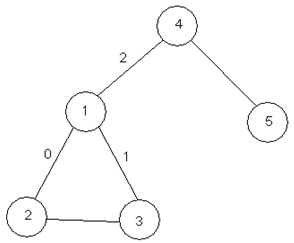# #254. 【Rujia Liu's Present 7】Explore the dune

### 交互格式

1. Look，表示你观察四周。
• 交互库会输出一行两个整数 $d,s$，其中 $d$ 表示当前洞穴连接道路条数，$s=0$ 表示当前洞穴没有路标，$s=1$ 表示当前洞穴有路标。
2. Walk i，其中 $i$ 是一个整数，表示走过当前洞穴连接着的道路 $i$。初始时洞穴连接的道路逆时针编号 $0,1,\cdots,d-1$，随机起点，而之后的每个洞穴以同样方式编号，但是保证道路 $0$ 是来时的道路，即除了第一次 Walk 操作，保证 Walk 0 是返回。
• 交互库不会返回任何信息。
3. Put 将路标放置在当前洞穴，你需要保证路标在身上。
• 交互库不会返回任何信息。
4. Take 表示将当前洞穴的路标拿起，你需要保证当前洞穴有路标。
• 交互库不会返回任何信息。
5. Answer n m，表示你认为这个迷宫有 $n$ 个洞穴和 $m$ 条道路。
• 交互库不会返回任何信息，并将结束这组数据的评测。

### 样例

1 $~$
$~$ Look
3 0 $~$
$~$ Put
$~$ Walk 0
$~$ Look
2 0 $~$
$~$ Walk 1
$~$ Look
2 0 $~$
$~$ Walk 1
$~$ Look
3 1 $~$
$~$ Take
$~$ Walk 1
$~$ Look
2 0 $~$
$~$ Walk 1
$~$ Look
1 0 $~$
$~$ Answer 5 5

#### explanation### 来源

Uva Online Judge，NOI2004，题目作者刘汝佳，贝小辉。